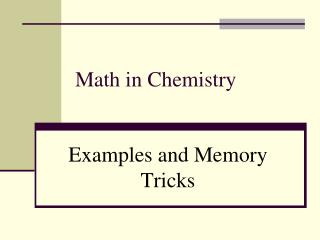DownloadDownload PresentationMath in Chemistry

# Math in Chemistry

Download Presentation## Math in Chemistry

- - - - - - - - - - - - - - - - - - - - - - - - - - - E N D - - - - - - - - - - - - - - - - - - - - - - - - - - -
##### Presentation Transcript

1. Math in Chemistry Examples and Memory Tricks

2. Limiting Reactants 79.1 g of zinc react with 0.90 L of HCl. Identify the limiting and excess reactants. How many liters of hydrogen are formed at STP? Zn + 2HCl  ZnCl2 + H2 79.1 g 0.90 L ? L

3. Limiting Reactants Zn + 2HCl  ZnCl2 + H2 79.1 g 0.90 L ? L 79.1 g Zn 1 mol Zn 65.39 g Zn 1 mol H2 1 mol Zn 22.4 L H2 1 mol H2 = 27.1 L H2

4. Limiting Reactants Zn + 2HCl  ZnCl2 + H2 79.1 g 0.90 L ? L 0.90 L HCl 1 mol HCl 22.4 L HCl 1 mol H2 2 mol HCL 22.4 L H2 1 mol H2 = 0.45 L H2

5. Limiting Reactants Zn: 27.1 L H2 HCl: 0.45 L H2 Limiting reactant: HCl Excess reactant: Zn

6. Percent Yield • When 45.8 g of K2CO3 react with excess HCl, 46.3 g of KCl are formed. Calculate the theoretical and % yields of KCl. K2CO3 + 2HCl  2KCl + H2O + CO2

7. Mass-Mass Stoichiometry The reaction of fluorine with ammonia produces dinitrogen tetrafluoride and hydrogen fluoride. 5F2 + 2 NH3→ N2F4 + 6HF 1. How many grams of NH3 are required to produce 7.38g HF? 2. How many grams of N2F4 can be produced from 265g F2?

8. 2C3H7OH + 9O2→ 6CO2 + 8H2O Mole-Mole Examples Isopropyl alcohol (C3H7OH) burns in the air to this equation: • Write the equation. • Calculate the mass of oxygen needed to react with 3.40 moles of isopropyl alcohol. • Find the moles of water when 6.20 mol O2 reacts with C3H7OH.

9. Formulas to Use Mole - Mole Known mol of unknown mol of known Mol Ratio – from equation Mass - Mass mol of unknown Known g 1 mol known molar mass unknown mol of known 1 mol unknown molar mass known OR OR Other 6.02 × 1023 particles mol of unknown Known L 1 mol known 22.4 L unknown OR mol of known 1 mol unknown particles 22.4 L known 6.02 × 1023 particles